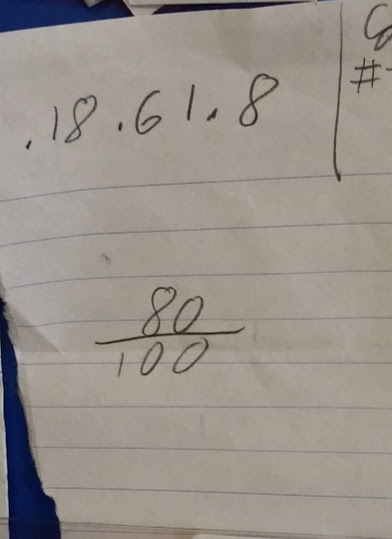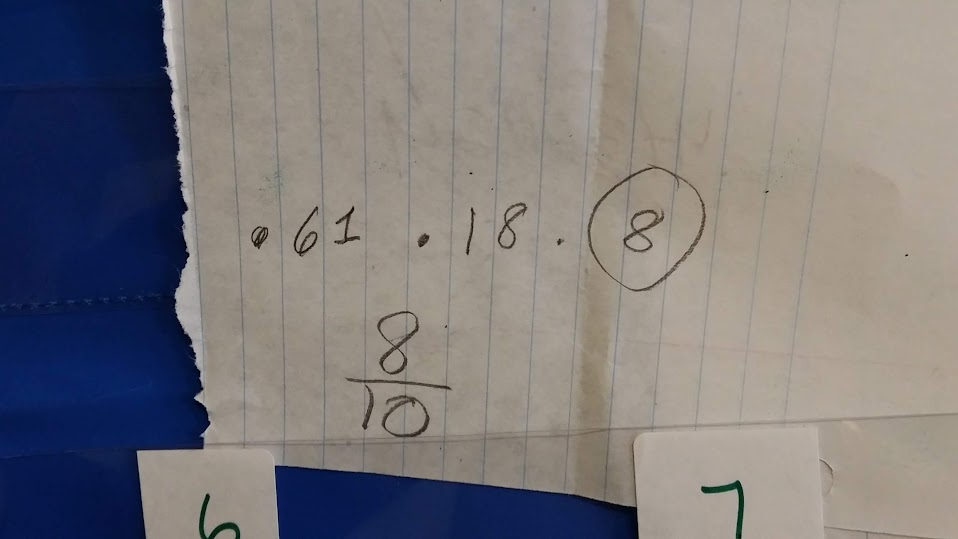# Smallest to Largest - Ordering Decimals

9 teachers like this lesson
Print Lesson

## Objective

SWBAT compare decimals and read and write decimals correctly to the hundredths place.

#### Big Idea

In this cooperative learning lesson, students play a math card game in order to compare and read decimals to the hundredths place.

## Warm Up

7 minutes

Students start today's lesson with a fluency assessment.  This assessment is from Monitoring Basic Skills Progress Second Edition: Basic Math Computation by Lynn S. Fuchs, Carol L. Hamlett, and Douglas Fuchs.

This is an assessment I have my students do each week and then graph their results.  It allows them to reflect on their learning of basic math facts, as well as using all four operations with whole numbers, and adding and subtracting unit fractions.  (It also happens to be the quietest time in my math classroom all week!!)

This is what my classroom looks like as students work on this assessment.

This is sample of a student's graph. You can also click here to hear my thoughts about this graph below.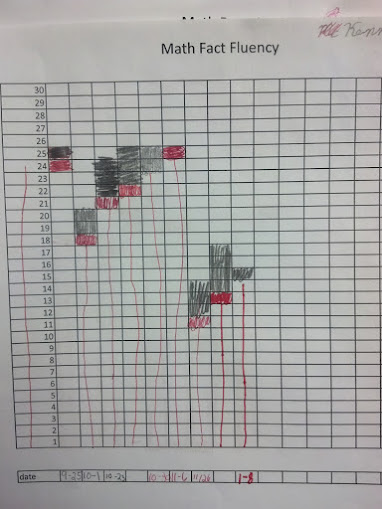Click hereto see an example of a typical fourth grade fluency decrease since students are completing the fourth grade fluency set that incorporates division, multiplication, addition, subtraction, and adding and subtracting fractions with like denominators. (At this point, students have not had many fraction lessons, thus very very students are able to complete the fraction problems)

I do not start my students with the fourth grade skills, but at this point in the year, all students are using the fourth grade set.. I chose to start them with the end of the third grade skills which covers addition, subtraction and multiplication and division of basic facts. I strongly believe in a balanced math approach, which is one reason why I also believe in common core standards.  By having a balance of building conceptual understanding, application of problems, and computational fluency, students can experience rigorous mathematics.  I want to make clear that this assessment ONLY measures basic math computation.  It is only one piece of students' knowledge. The assessments in this book, for each grade level, do not change in difficulty over the course of the year.  Therefore, a student's increase in score over the school year truly reflects improvement in the student's ability to work the math problems at that grade level.

## Concept Development

50 minutes

I begin this lesson by telling students that they will practice comparing decimals to the hundredths place today.  I ask students to write down 10 numbers that come between 3.1 and 3.4 on their whiteboards.  I encourage students to use base ten blocks to model the numbers especially if they are stuck.  (I know several students will only think of 2 numbers, 3.2 and 3.3.  Therefore, I have base ten blocks ready at each table for students to model these numbers)

The process of comparing decimal numbers is actually exactly the same process for comparing whole numbers, although students might not realize this at first. Instead of focusing on place value, students look at the 'length' of the whole numbers, and hence base their perception of the relative size of whole numbers on the relative number of digits involved. For example, they might say that "1576 is larger than 742 as it is contains more digits" or "1576 is bigger than 742 as it is longer". While this strategy will provide correct answers for whole numbers, this lack of focus on place value is likely to lead to similar (but incorrect) strategies for comparing decimal numbers. For example, some students think that long decimals are usually large numbers, and surprisingly, some think that long decimals are usually small numbers.

After about 7 minutes, I ask students to stop working and share their numbers with their learning partner. As students share, I circulate around the room and listen to students thinking about how they knew what numbers to write down.

In this video, you can see a student has written down some correct numbers that fall between 3.1 and 3.4.  Notice that he does say that one of his table mates helped him and told him that he could think about 3.1 and 3.10 and 3.4 as 3.40 to think about numbers between.  I love how comfortable my students are helping each other. I have worked hard to set up a climate of math talk and student collaboration and this student example was a great way to see that.

Next, I introduce students to a game called Smaller to Larger.

Materials needed to play the game are a deck of cards with Jacks, and Queens removed, piece of paper per student, and a pencil. For this game today, I ask students to play in groups of three, however the game can be played in partners and groups of four as well.  I choose to use groups of three because my students spend most days working with partners.  Working in groups of three is a change of pace that keeps students engaged.

To play the game:

1. Students begin by drawing a large three by three grid on their piece of paper.

2. Next students place the deck of cards face down between all players. (Kings have a value of 0 for this game)

3.  Players then take turns drawing two cards.  On their turn, the first card drawn is the tenths, and the second card drawn is the hundredths.

4.  Students then place their decimal on an empty square on their game board.  They must place the decimal so that all rows will be arranged from smallest to largest, and all columns will be arranged smallest to largest.  Students should place the played decimal cards in a discard pile.

5. If students make a decimal that they can not place on their board, they keep those two cards and lose their turn.

6. The game is over when EACH player has their board filled.  The person with the least amount of cards is the winner.

In this video, students are playing the smallest to largest game. Notice how the girl uses the correct terminology when reading the decimal.  Also, this group misunderstood the directions and began creating a largest to smallest board instead of smallest to largest.  Since the purpose for this game was to read, write, and order decimals, I allowed the group to continue with largest to smallest.

In this video, two other students are playing the smallest to largest game.  You can hear one student expressing some hesitation about how to read her decimal and wrestling with where to place it on her board.  This was a great reminder to me that this game is so important for students to practice reading decimals as well as making sense of their sizes.

## Check for Understanding

5 minutes

To end this lesson, I direct students to complete an exit ticket.  You can see in the photo below the question I pose to students.  I ask students to order the three decimal numbers from smallest to largest and then circle the largest decimal and also record that number as a fraction.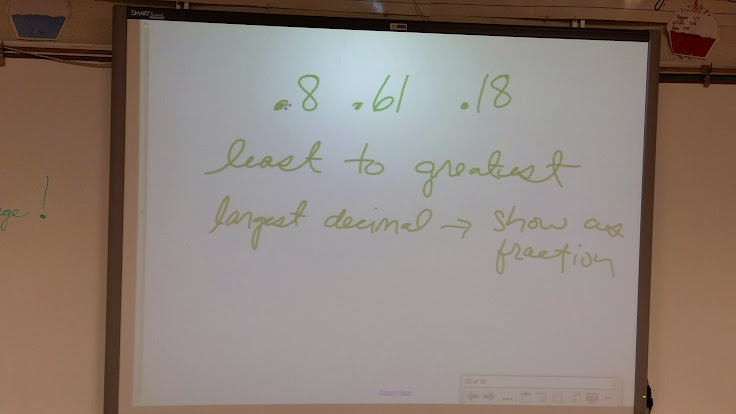This exit ticket is important because it allows me to see how students are progressing with CCSS 4.NF.6 (Use decimal notation for fractions with denominators 10 or 100. For example, rewrite 0.62 as 62/100; describe a length as 0.62 meters; locate 0.62 on a number line diagram.) and CCSS 4.NF.7 (Compare two decimals to hundredths by reasoning about their size. Recognize that comparisons are valid only when the two decimals refer to the same whole. Record the results of comparisons with the symbols >, =, or <, and justify the conclusions, e.g., by using a visual model.)  By viewing and analyzing the results I can design future lesson and learning opportunities for students.

When students are finished with the problem they place their exit ticket in an exit ticket holder I have hanging on my classroom door. This helps me keep their work organized and serves a quick way for me to glance and see if everyone has turned a ticket in.  Each of my students are assigned a number and they place their exit ticket in the pocket that corresponds to their number.  In the photo below, you can see an example of my exit ticket holder.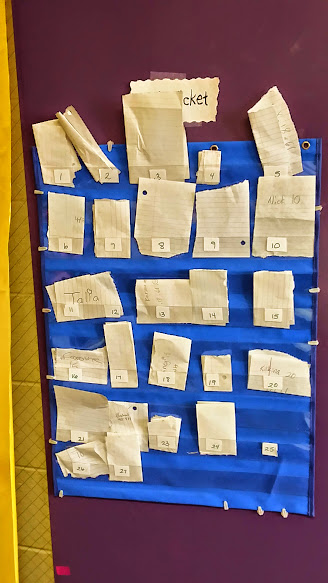Notice in these two exit tickets,  that both student have identified .8 as the largest decimal. One student then writes the fraction as 80/100 and the other writes it as 8/10.  This will lead to a great discussion in future math classes.  You can see that the students work on the right does not have the decimals ordered correctly from least to greatest. I am hoping that tomorrows lesson will also help this student deepen his/her understanding of comparing and ordering decimals to the hundredths place, but I will be keeping a close eye on this student to make sure he understands this concept.